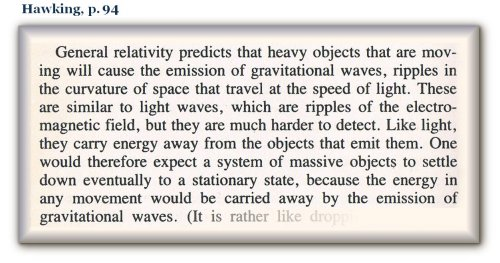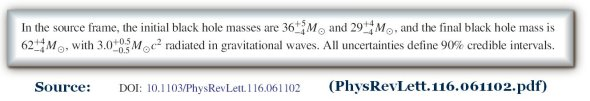What is the source of gravitational energy?

 1 2 3 4 5 6 7 8 9 10
 2 Is it velocity or is it mass that supplies the energy source for gravitational-waves? Let us return to Hawking's definition of gravitational-waves:So the source energy for the gravitational-waves is supposedly the velocity of the objects. It would therefore be quite a surprise if someone were to claim that the source for the energy of the gravitational-waves is not the velocity, but the mass of the object. And yet, that is what Abbot is claiming:.
 No less than the amount of mass of three suns is supposedly emitted as energy for the interaction of 65 suns’ worth; even though the amount of energy supposedly emitted by the Earth as a result of its interaction with the Sun is said by Hawking to be:So if the Earth is losing the energy of an electric heater, then the pair of black-holes would account for about 20 million electric heaters worth of energy. This is about as much energy as the usage of an ordinary city for GW150914. So how does a city’s worth of energy compare with the amount of energy contained in all the mass of three suns multiplied by the velocity of light squared? Wikipedia (October 2016) had this to say:.

 Next Page ->

^ Top of Page ^